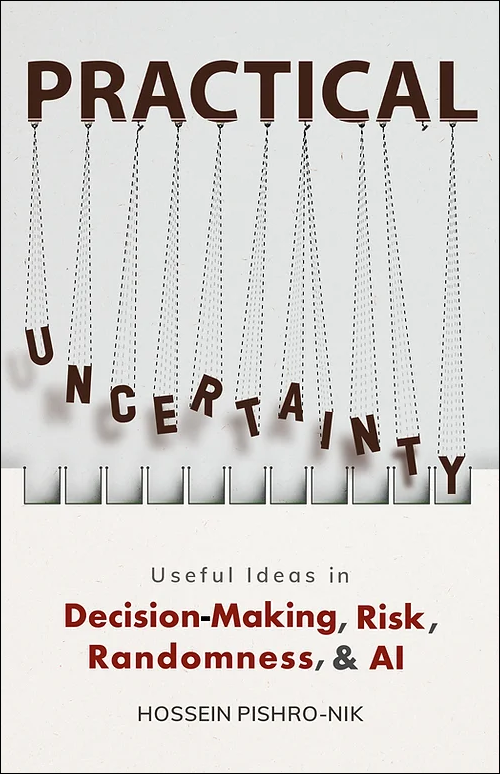## 4.1.0 Continuous Random Variables and their Distributions

We have in fact already seen examples of continuous random variables before, e.g., Example 1.14. Let us look at the same example with just a little bit different wording.

Example

I choose a real number uniformly at random in the interval $[a,b]$, and call it $X$. By uniformly at random, we mean all intervals in $[a,b]$ that have the same length must have the same probability. Find the CDF of $X$.

• Solution
• As we mentioned, this is almost exactly the same problem as Example 1.14, with the difference being, in that problem, we considered the interval from $1$ to $2$. In that example, we saw that all individual points have probability $0$, i.e., $P(X=x)=0$ for all $x$. Also, the uniformity implies that the probability of an interval of length $l$ in $[a,b]$ must be proportional to its length: $$P(X \in [x_1,x_2]) \propto (x_2-x_1), \hspace{20pt} \textrm{where }a \leq x_1 \leq x_2 \leq b.$$ Since $P(X \in [a,b])=1$, we conclude $$P(X \in [x_1,x_2]) =\frac{x_2-x_1}{b-a}, \hspace{20pt} \textrm{where }a \leq x_1 \leq x_2 \leq b.$$ Now, let us find the CDF. By definition $F_X(x)=P(X \leq x)$, thus we immediately have $$F_X(x) =0, \hspace{20pt} \textrm{for } x < a,$$ $$F_X(x) =1, \hspace{20pt} \textrm{for } x \geq b.$$ For $a \leq x \leq b$, we have  $F_X(x)$ $=P(X \leq x)$ $=P(X \in [a,x])$ $=\frac{x-a}{b-a}.$

Thus, to summarize \begin{equation} \hspace{70pt} F_X(x) = \left\{ \begin{array}{l l} 0 & \quad \textrm{for } x < a \\ \frac{x-a}{b-a} & \quad \textrm{for }a \leq x \leq b\\ 1 & \quad \textrm{for } x > b \end{array} \right. \hspace{70pt} (4.1) \end{equation} Note that here it does not matter if we use "$<$" or "$\leq$", as each individual point has probability zero, so for example $P(X < 2)=P(X \leq 2)$. Figure 4.1 shows the CDF of $X$. As we expect the CDF starts at zero and ends at $1$.Fig.4.1 - CDF for a continuous random variable uniformly distributed over $[a,b]$.

One big difference that we notice here as opposed to discrete random variables is that the CDF is a continuous function, i.e., it does not have any jumps. Remember that jumps in the CDF correspond to points $x$ for which $P(X=x) > 0$. Thus, the fact that the CDF does not have jumps is consistent with the fact that $P(X=x)=0$ for all $x$. Indeed, we have the following definition for continuous random variables.

Definition
A random variable $X$ with CDF $F_X(x)$ is said to be continuous if $F_X(x)$ is a continuous function for all $x \in \mathbb{R}$.

We will also assume that the CDF of a continuous random variable is differentiable almost everywhere in $\mathbb{R}$.

 The print version of the book is available on Amazon.Practical uncertainty: Userful Ideas in Decision-Making, Risk, Randomness, & AI